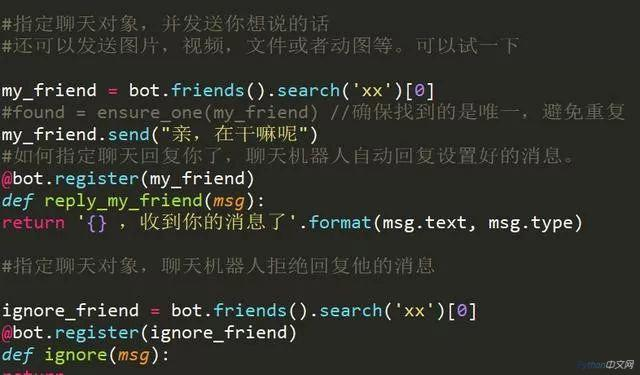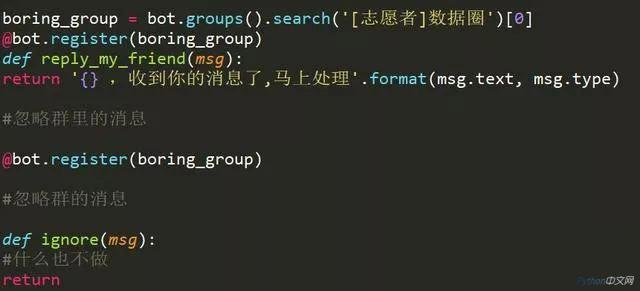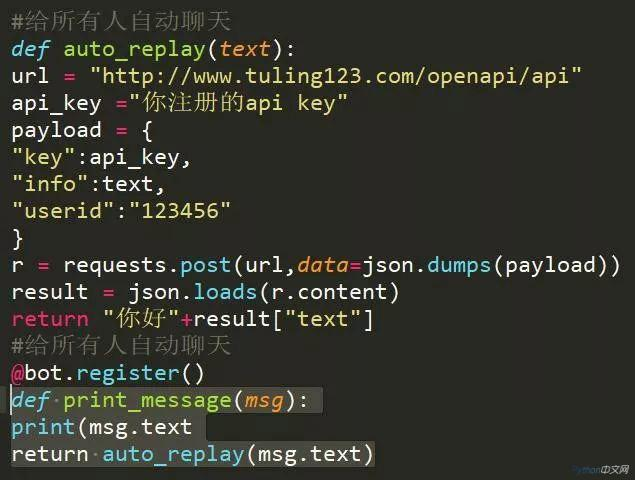# 520情人节！教你用Python给自己造了一个女朋友• 小P   2019-05-20 14:01:221048

520这一天，也是网络情人节。就在那天，如果没有女朋友的自己，如何假装自己是有朋友的！如何在朋友圈“秀恩爱”• 运行平台：Mac

• Python版本：Python3.6

• IDE：spyder

• 模块：wxpy（pip install wxpy）Python

520表白神器！心爱的她

```from turtle import *
from time import sleep

def go_to(x, y):
up()
goto(x, y)
down()

def big_Circle(size):  #函数用于绘制心的大圆
speed(1)
for i in range(150):
forward(size)
right(0.3)

def small_Circle(size):  #函数用于绘制心的小圆
speed(1)
for i in range(210):
forward(size)
right(0.786)

def line(size):
speed(1)
forward(51*size)

def heart( x, y, size):
go_to(x, y)
left(150)
begin_fill()
line(size)
big_Circle(size)
small_Circle(size)
left(120)
small_Circle(size)
big_Circle(size)
line(size)
end_fill()

def arrow():
pensize(10)
go_to(-400, 0)
left(15)
forward(150)
go_to(339, 178)
forward(150)

pensize(1)
speed(1)
color('red', 'red')
begin_fill()
left(120)
forward(20)
right(150)
forward(35)
right(120)
forward(35)
right(150)
forward(20)
end_fill()

def main():
pensize(2)
color('red', 'pink')
#getscreen().tracer(30, 0) #取消注释后，快速显示图案
heart(200, 0, 1)          #画出第一颗心，前面两个参数控制心的位置，函数最后一个参数可控制心的大小